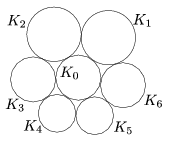Mathematical and Physical Journal
for High Schools
Issued by the MATFUND Foundation
 Already signed up? New to KöMaL?

#Problem A. 507. (April 2010)

A. 507. The circles $\displaystyle K_1,\dots,K_6$ are externally tangent to the circle $\displaystyle K_0$ in this order. For each $\displaystyle 1\le i\le 5$, the circles $\displaystyle K_i$ and $\displaystyle K_{i+1}$ are externally tangent to each other, and $\displaystyle K_1$ and $\displaystyle K_6$ are externally tangent to each other as well, according to the Figure. Denote by $\displaystyle r_i$ the radius of $\displaystyle K_i$ ($\displaystyle 0\le i\le6$). Prove that if $\displaystyle r_1r_4=r_2r_5=r_3r_6=1$ then $\displaystyle {r_0\le 1}$.Proposed by: Balázs Strenner, Székesfehérvár

(5 pont)

Deadline expired on May 10, 2010.

### Statistics:

 2 students sent a solution. 4 points: Nagy 648 Donát. 1 point: 1 student.

Problems in Mathematics of KöMaL, April 2010